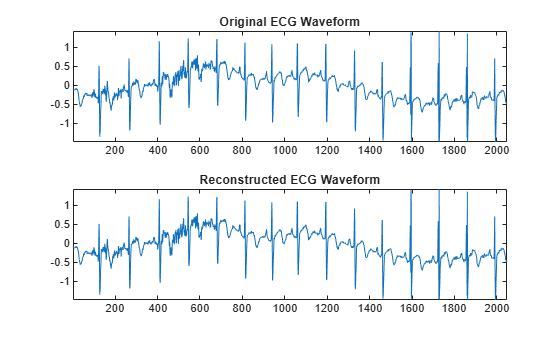imodwpt

Inverse maximal overlap discrete wavelet packet transform

Description

example

xrec = imodwpt(coefs) returns the inverse maximal overlap discrete wavelet packet transform (inverse MODWPT), in xrec. The inverse transform is for the terminal node coefficient matrix (coefs) obtained using modwpt with the default length 18 Fejér-Korovkin ('fk18') wavelet.

example

xrec = imodwpt(coefs,wname) returns the inverse MODWPT using the orthogonal filter specified by wname. This filter must be the same filter used in modwpt.

example

xrec = imodwpt(coefs,lo,hi) returns the inverse MODWPT using the orthogonal scaling filter, lo, and wavelet filter, hi.

Examples

collapse all

Obtain the MODWPT of an ECG waveform and demonstrate perfect reconstruction using the inverse MODWPT.

wpt = modwpt(wecg);
xrec = imodwpt(wpt);
subplot(2,1,1)
plot(wecg);
title('Original ECG Waveform');
subplot(2,1,2)
plot(xrec);
title('Reconstructed ECG Waveform');Find the largest absolute difference between the original signal and the reconstruction. The difference is on the order of $1{0}^{-11}$, which demonstrates perfect reconstruction.

max(abs(wecg-xrec'))
ans = 1.7903e-11

Obtain the MODWPT of Southern Oscillation Index data using the Daubechies extremal phase wavelet with two vanishing moments ('db2'). Reconstruct the signal using the inverse MODWPT.

wsoi = modwpt(soi,'db2');
xrec = imodwpt(wsoi,'db2');

Obtain the MODWPT of Southern Oscillation Index data using specified scaling and wavelets filters with the Daubechies extremal phase wavelet with two vanishing moments ('db2').

[lo,hi] = wfilters('db2');
wpt = modwpt(soi,lo,hi);
xrec = imodwpt(wpt,lo,hi);

Plot the original SOI waveform and the reconstructed waveform.

subplot(2,1,1)
plot(soi)
title('Original SOI Waveform');
subplot(2,1,2)
plot(xrec)
title('Reconstructed SOI Waveform')Input Arguments

collapse all

Terminal node coefficients of a wavelet packet tree, specified as a matrix. You must obtain the coefficient matrix from modwpt using the 'FullTree',false option. 'FullTree',false is the default value of modwpt.

Data Types: double

Synthesizing wavelet filter used to invert the MODWPT, specified as a character vector or string scalar. The specified wavelet must be the same wavelet as used in the analysis with modwpt.

Scaling filter, specified as an even-length real-valued vector. lo must be the same scaling filter as used in the analysis with modwpt. You cannot specify both a scaling-wavelet filter pair and a wname filter.

Wavelet filter, specified as an even-length real-valued vector. hi must be the same wavelet filter used in the analysis with modwpt. You cannot specify both a scaling-wavelet filter pair and a wname filter.

Output Arguments

collapse all

Inverse maximal overlap discrete wavelet packet transform, returned as a row vector. The inverse transform is the reconstructed version of the original signal based on the MODWPT terminal node coefficients. xrec has the same number of columns as the input coefs matrix.

 Percival, D. B., and A. T. Walden. Wavelet Methods for Time Series Analysis. Cambridge, UK: Cambridge University Press, 2000.

 Walden, A.T., and A. Contreras Cristan. “The phase-corrected undecimated discrete wavelet packet transform and its application to interpreting the timing of events.” Proceedings of the Royal Society of London A. Vol. 454, Issue 1976, 1998, pp. 2243-2266.

Wavelet Toolbox DocumentationGet trial now